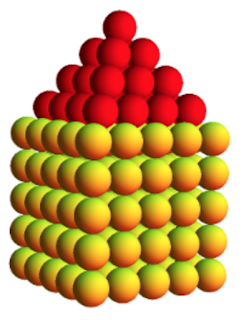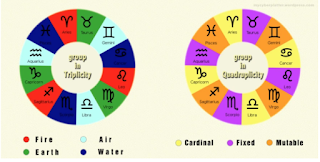Tuesday, 29 December 2020

Prime 2-D House Numbers

I made a post on Friday, 14th February 2020, titled House Numbers that were three-dimensional figurate numbers that looked like houses (see Figure 1).Figure 1

These numbers were characterised by two conjoined shapes: a cube, on top of which sits a square-based pyramid. The sequence of house numbers forms OEIS A051662. Today I turned 26203 days old and this turns out to be a member of another sequence, namely OEIS A229080.

 Primes of the form $T_n$ + $S_n$ + $1$ where $T_n$ is the $n$-th triangular number and $S_n$ is the $n$-th square number.

The second member of the sequence is 41 and it can be represented as shown in Figure 2:Figure 2

It struck me that all such numbers in this sequence could be represented in this way with the square representing the body of the house, the triangle the roof and with "a cherry on top" so to speak. The "cherry" is the number 1 that must be added to triangular and square components to produce a prime (in some cases). The prime is of the form:$$n^2+\frac{n \, (n+1)}{2}+1=\frac{3n^2+n+2}{2} \text{ where } n \geq 1$$The situation with 26203 is shown in Figure 3.Figure 3

These numbers remind me of 2-D versions of the house numbers in my earlier post and hence my choice for their description: prime 2-D house numbers. Not every sum of a square number, triangular number and 1 will produce a prime of course. In the case of $n=2$, the total is 4 + 3 + 1 = 8 which is not prime and is the only value of $n$ for which the sum of the square (4) and the triangle (3) is a prime.

All this of course is primary level Mathematics but part of the reason that I made this post was that the same day that had my square (4) and triangle (3) number references, I had started to read David Lindorff's "Pauli and Jung: The Meeting of Two Great Minds". In his book, the author writes:

A key to this scientific achievement was his recognition that the electrons must satisfy four quantum numbers rather than three, as had been previously assumed. It is beyond our scope to elaborate on the meaning of "quantum number" except to say that it relates to the electron's allowable energy states in an atom. The fourth quantum number was identified with what has been called an electron spin. In alchemy as well as in Jung's psychology, moving from three to four symbolises a completion, or a movement toward the centre. The alchemists identified their magnum opus with a fourfold process, which was symbolised by the so-called Axiom of Maria: one becomes two, two becomes three, and three becomes four as the one. ln association with modern dreams, Jung saw movement from three to four as symbolising a stage of inner development known as the individuation process. Pauli saw his discovery of the exclusion principle in that light.

Such a coincidence regarding threes and fours was described by Jung as synchronicity and he wrote a book titled "Synchronicity: An Acausal Connecting Principle". These types of numbers (primes that are the sum of a triangular and square number with a 1 added) are quite rare. For example, the previous such number was 21661 and the next such number will be 31177. Yet despite the rarity, this three and four related number popped up on the same day that I read the paragraph above.

Such synchronicity helps to remind me of the numinous nature of three and four and how we use such numbers daily without understanding the bottomless depths of three-ness and four-ness. The mandala is a symbol of wholeness usually encompassing in its structure the unfolding of that unity through two-fold, three-fold, four-fold division and beyond. The typical Western astrological chart is perhaps the best-known mandala and emphasises the numbers:
• 2 representing duality through:
• the dyads or signs that are diametrically opposite in the zodiac such as Aries and Libra
• the division of the chart into above the horizon and below the horizon houses
• the division of the chart into the eastern and western hemispheres
• the opposition aspect of 180°, being 360° divided by 2
• 3 representing harmony through:
• the three signs associated with each of the four triplicities of fire, earth, air and water
• the trine aspect of 120°, being 360° divided by 3
• 4 representing foundation through:
• the four signs associated with each of three quadruplicities of cardinal, fixed and mutable
• the square aspect of 90°, being 360° divided by 4
• the four sections of the chart produced by the division of the circle by the Ascendant-Descendant axis and MC-IC axis
The numbers 5, 6, 8, 9, 10 and 12 are also represented of course but I won't go into further details here about that. Figure 4 shows the triplicities and quadruplicities of the astrological chart.Figure 4

So let's not pretend we understand these numbers and archetypal significance. We don't. We never will.